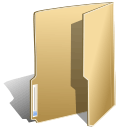## Objects (14)

Tuesday, 27 August 2019 07:22

### Add arrow line to Excel worksheet in C#

Written by support iceblue

With Spire.XLS for .NET, developers can easily use C# to add shapes to Excel worksheet. From version 9.8.11, Spire.XLS supports to add arrow lines to Excel worksheet. The following sample will show you how to insert arrow line, double Arrow, Elbow Arrow, Elbow Double-Arrow, Curved Arrow and Curved Double-Arrow to Excel worksheet in C#.

```using Spire.Xls;
using System.Drawing;

{
class Program
{
static void Main(string[] args)
{
//Initiate a Workbook object and get the first worksheet
Workbook workbook = new Workbook();
Worksheet sheet = workbook.Worksheets;

//Add a Double Arrow and fill the line with solid color
line.Top = 10;
line.Left = 20;
line.Width = 100;
line.Height = 0;
line.Color = Color.Blue;

//Add an Arrow and fill the line with solid color
line_1.Top = 50;
line_1.Left = 30;
line_1.Width = 100;
line_1.Height = 100;
line_1.Color = Color.Red;

line3.LineShapeType = LineShapeType.ElbowLine;
line3.Width = 30;
line3.Height = 50;
line3.Top = 100;
line3.Left = 50;

line2.LineShapeType = LineShapeType.ElbowLine;
line2.Width = 50;
line2.Height = 50;
line2.Left = 120;
line2.Top = 100;

line3.LineShapeType = LineShapeType.CurveLine;
line3.Width = 30;
line3.Height = 50;
line3.Top = 100;
line3.Left = 200;

line2.LineShapeType = LineShapeType.CurveLine;
line2.Width = 30;
line2.Height = 50;
line2.Left = 250;
line2.Top = 100;

//Save the file

}
}
}
```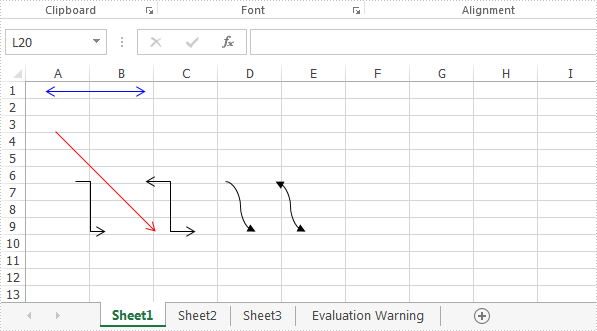Friday, 22 March 2019 07:11

### Set the internal margin of excel textbox in C#

Written by support iceblue

With Spire.XLS, developers can add text or image to the textbox to Excel worksheet easily. From version 9.3.10, Spire.XLS supports to set the inner margin of contents on Excel text box. With this feature, we can adjust the position of the text contents on the textbox to make it beautiful. This article is going to introduce how to set the inner margins of the textbox in Excel worksheet in C#.

```{

Workbook workbook = new Workbook();

//get the first worksheet
Worksheet sheet = workbook.Worksheets;

//add a textbox to the sheet and set its position and size
XlsTextBoxShape textbox = sheet.TextBoxes.AddTextBox(4, 2, 100, 300) as XlsTextBoxShape;

//set the text on the textbox
textbox.Text = "Insert TextBox in Excel and set the margin for the text";
textbox.HAlignment = CommentHAlignType.Center;
textbox.VAlignment = CommentVAlignType.Center;

//set the inner margins of the contents
textbox.InnerLeftMargin = 1;
textbox.InnerRightMargin = 3;
textbox.InnerTopMargin = 1;
textbox.InnerBottomMargin = 1;

//save the document to file
workbook.SaveToFile("Result.xlsx", ExcelVersion.Version2010);

}
```

Effective screenshot after setting the margins of the contents: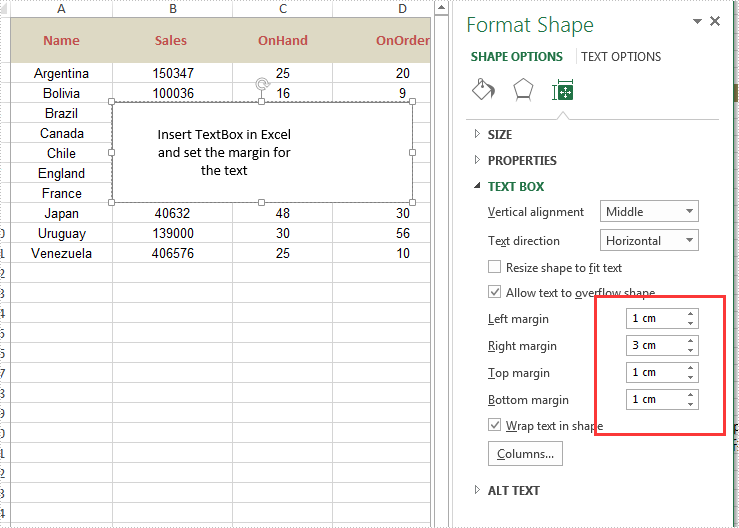Wednesday, 30 May 2018 07:57

### Hide or Unhide Shape in Excel in C#

Written by support iceblue

Spire.XLS supports to hide or unhide certain shapes in Excel worksheet through IShape.Visible property. This article demonstrates the detail steps to hide or unhide a shape using Spire.XLS and C#.

Below is the screenshot of the example Excel file: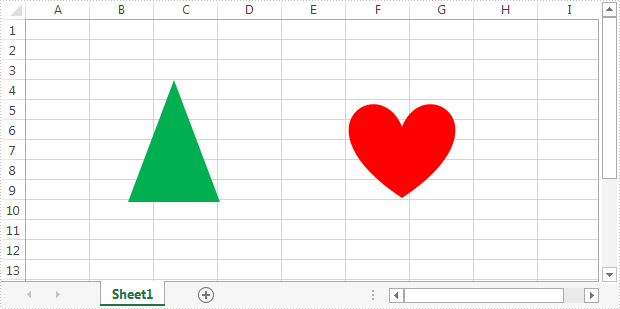Detail steps:

Step 1: Instantiate a Workbook object and load the Excel file.

```Workbook workbook = new Workbook();
```

Step 2: Get the first worksheet.

```Worksheet sheet = workbook.Worksheets;
```

Step 3: Hide the second shape in the worksheet.

```//Hide the second shape in the worksheet
sheet.PrstGeomShapes.Visible = false;

//Show the second shape in the worksheet
//sheet.PrstGeomShapes.Visible = true;
```

Step 4: Save the file.

```workbook.SaveToFile("HideShape.xlsx", ExcelVersion.Version2013);
```

Output: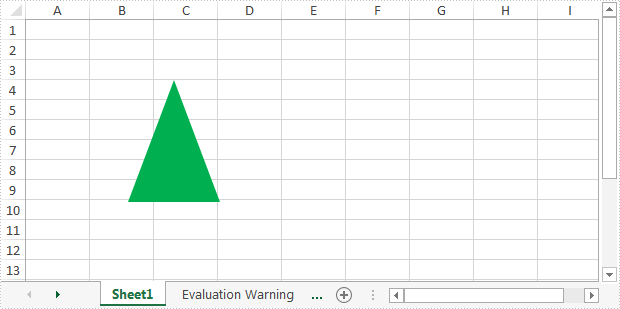Full code:

```//Instantiate a Workbook object
Workbook workbook = new Workbook();

//Get the first worksheet
Worksheet sheet = workbook.Worksheets;

//Hide the second shape in the worksheet
sheet.PrstGeomShapes.Visible = false;

//Show the second shape in the worksheet
//sheet.PrstGeomShapes.Visible = true;

//Save the file
workbook.SaveToFile("HideShape.xlsx", ExcelVersion.Version2013);
```
Wednesday, 03 January 2018 08:38

### Delete shapes in an Excel Worksheet in C#

Written by support iceblue

Spire.XLS supports to delete a specific shape as well as all shapes in an Excel worksheet. This article demonstrates how to use Spire.XLS to implement this function.

The example file we used for demonstration: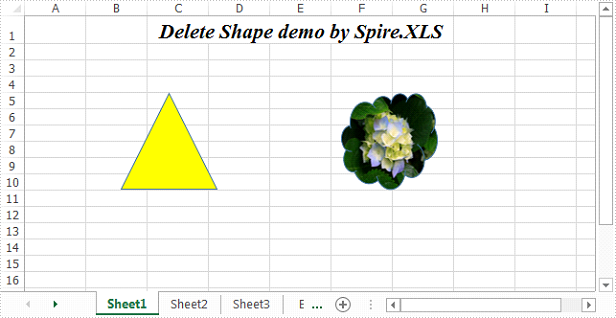Detail steps:

Step 1: Initialize an object of Workbook class and load the Excel file.

```Workbook workbook = new Workbook();
```

Step 2: Get the first worksheet.

```Worksheet sheet = workbook.Worksheets;
```

Step 3: Delete the first shape in the worksheet.

```sheet.PrstGeomShapes.Remove();
```

To delete all shapes from the worksheet:

```for (int i = sheet.PrstGeomShapes.Count-1; i >= 0; i--)
{
sheet.PrstGeomShapes[i].Remove();
}
```

Step 4: Save the file.

```workbook.SaveToFile("DeleteShape.xlsx", ExcelVersion.Version2013);
```

Screenshot: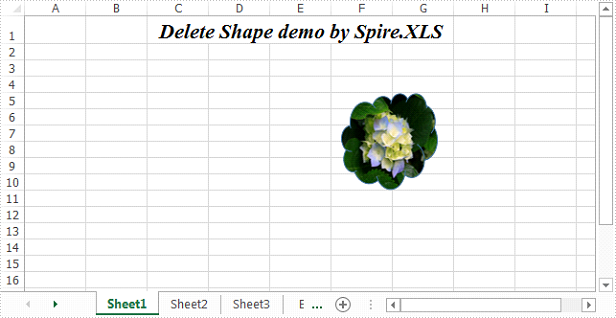Full code:

```//Initialize an object of Workbook class
Workbook workbook = new Workbook();

//Get the first worksheet
Worksheet sheet = workbook.Worksheets;

//Delete the first shape in the worksheet
sheet.PrstGeomShapes.Remove();

//Delete all shapes in the worksheet
//for (int i = sheet.PrstGeomShapes.Count-1; i >= 0; i--)
//{
//    sheet.PrstGeomShapes[i].Remove();
//}

workbook.SaveToFile("DeleteShape.xlsx", ExcelVersion.Version2013);
```
Tuesday, 19 December 2017 08:05

### Extract text and image from Excel shape in C#

Written by support iceblue

An excel shape can be filled with text or image, sometimes we need to read the text and image information in the shape. In this article, we are going to introduce how to extract text and image from shapes in Excel using Spire.XLS and C#.

Below is the screenshot of the sample document we used for demonstration: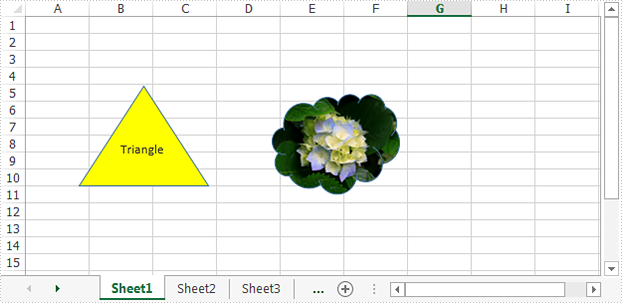Detail steps:

Step 1: Initialize an object of Workbook class and Load the Excel file.

```Workbook workbook = new Workbook();
```

Step 2: Get the first worksheet.

```Worksheet sheet = workbook.Worksheets;
```

Step 3: Extract text from the first shape and save to a txt file.

```IPrstGeomShape shape1 = sheet.PrstGeomShapes;
string s = shape1.Text;
StringBuilder sb = new StringBuilder();
sb.AppendLine(s);
File.WriteAllText("ShapeText.txt", sb.ToString());
```

Step 4: Extract image from the second shape and save to a local folder.

```IPrstGeomShape shape2 = sheet.PrstGeomShapes;
Image image = shape2.Fill.Picture;
image.Save(@"Image\ShapeImage.png", ImageFormat.Png);
```

Screeshot:

Extracted text: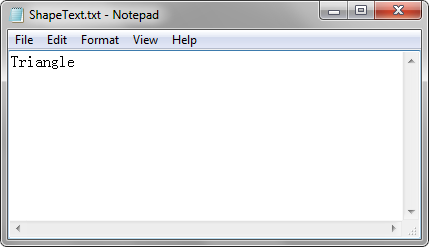Extracted image: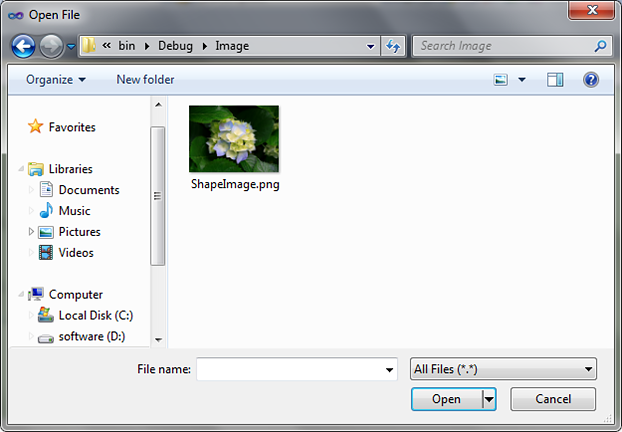Full code:

```using System.Drawing;
using System.Drawing.Imaging;
using System.IO;
using System.Text;
using Spire.Xls;
using Spire.Xls.Core;

namespace Extract_text_and_image_from_Excel_shape
{
class Program
{
static void Main(string[] args)
{
Workbook workbook = new Workbook();

//Get the first worksheet
Worksheet sheet = workbook.Worksheets;

//Extract text from the first shape and save to a txt file
IPrstGeomShape shape1 = sheet.PrstGeomShapes;
string s = shape1.Text;
StringBuilder sb = new StringBuilder();
sb.AppendLine(s);
File.WriteAllText("ShapeText.txt", sb.ToString());

//Extract image from the second shape and save to a local folder
IPrstGeomShape shape2 = sheet.PrstGeomShapes;
Image image = shape2.Fill.Picture;
image.Save(@"Image\ShapeImage.png", ImageFormat.Png);
}
}
}
```
Tuesday, 19 December 2017 07:39

### Set the shadow style for the shape on Excel worksheet in C#

Written by support iceblue

With the help of Spire.XLS, we can easily add shapes to the Excel worksheet. This article will demonstrate how to format the shadow of shape on Excel in C#. We can use Spire.XLS to set the color, size, blur, angle, transparency and distance of the shadow for the shape on Excel worksheet.

Set the shadow style when we add new shape to the Excel worksheet:

```//instantiate a Workbook object and get the first worksheet
Workbook workbook = new Workbook();
Worksheet sheet = workbook.Worksheets;

IPrstGeomShape ellipse = sheet.PrstGeomShapes.AddPrstGeomShape(5, 5, 150, 100, PrstGeomShapeType.Ellipse);

//set the shadow style for the ellipse

//save the document to file
```

Effective screenshot after setting the shadow style for the shape on the Excel worksheet: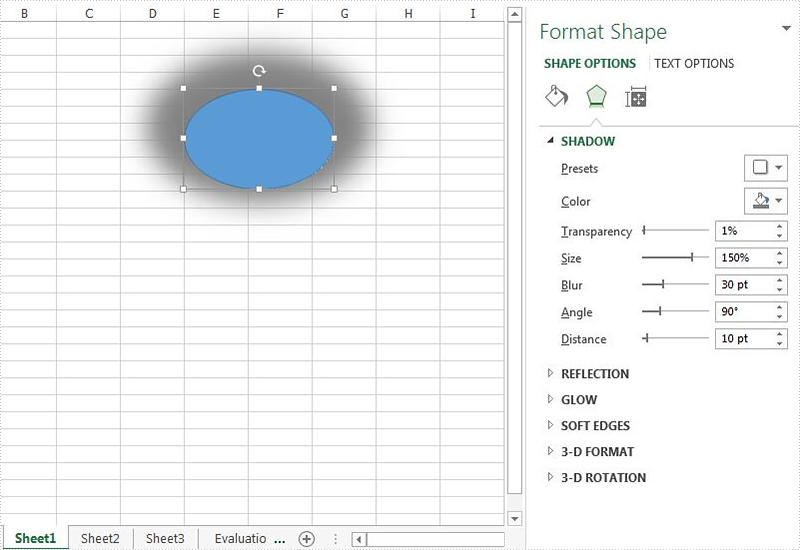Set the shadow style when we loaded an Excel document with shape:

```//create an instance of workbook and load the document from file
Workbook workbook = new Workbook();

//get the first worksheet from the sample document
Worksheet sheet = workbook.Worksheets;

//get the first shape from the worksheet
IPrstGeomShape shape = sheet.PrstGeomShapes;

//set the shadow style for the shape

//save the document to file
```

Effective screenshot after setting the shadow style for the shape on the Excel worksheet: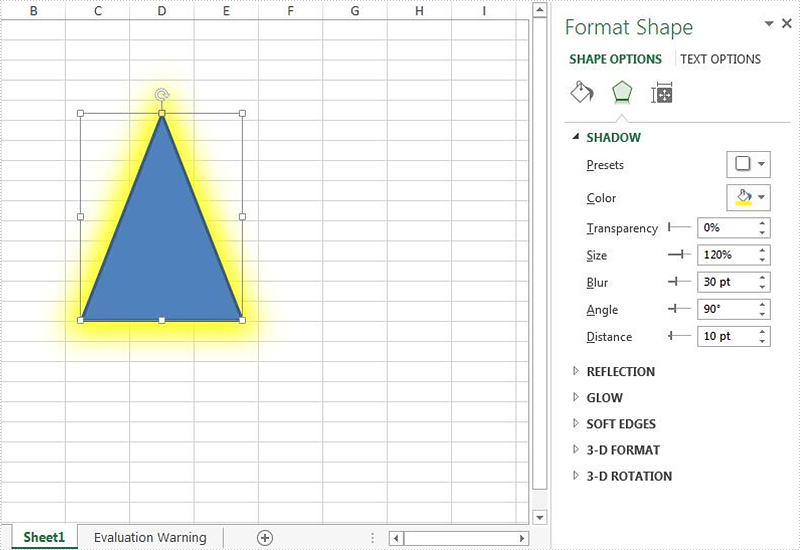Tuesday, 10 October 2017 07:34

### Insert Shapes to Excel Worksheet in C#

Written by support iceblue

Spire.XLS supports to add up to 186 kinds of ready-made shapes, such as triangles, arrows and callouts to Excel worksheet. This article demonstrates how to insert shapes to excel worksheet and fill the shapes with color and picture using Spire.XLS and C#.

Detail steps:

Step 1: Instantiate a Workbook object and get the first worksheet.

```Workbook workbook = new Workbook();
Worksheet sheet = workbook.Worksheets;
```

Step 2: Add a triangle shape and fill the shape with solid color.

```//Add a triangle shape
IPrstGeomShape triangle = sheet.PrstGeomShapes.AddPrstGeomShape(2, 2, 100, 100, PrstGeomShapeType.Triangle);

//Fill the triangle with solid color
triangle.Fill.ForeColor = Color.Yellow;
triangle.Fill.FillType = ShapeFillType.SolidColor;
```

Step 3: Add a heart shape and fill the shape with gradient color.

```//Add a heart shape
IPrstGeomShape heart = sheet.PrstGeomShapes.AddPrstGeomShape(2, 5, 100, 100, PrstGeomShapeType.Heart);

//Fill the heart with gradient color
heart.Fill.ForeColor = Color.Red;
```

Step 4: Add an arrow shape with default color.

```IPrstGeomShape arrow = sheet.PrstGeomShapes.AddPrstGeomShape(10, 2, 100, 100, PrstGeomShapeType.CurvedRightArrow);
```

Step 5: Add a cloud shape and fill the shape with custom picture.

```//Add a cloud shape
IPrstGeomShape cloud = sheet.PrstGeomShapes.AddPrstGeomShape(10, 5, 100, 100, PrstGeomShapeType.Cloud);

//Fill the cloud with picture
cloud.Fill.CustomPicture(Image.FromFile("Hydrangeas.jpg"), "Hydrangeas.jpg");
cloud.Fill.FillType = ShapeFillType.Picture;
```

Step 6: Save the file.

```workbook.SaveToFile("AddShapes.xlsx", ExcelVersion.Version2013);
```

Screenshot: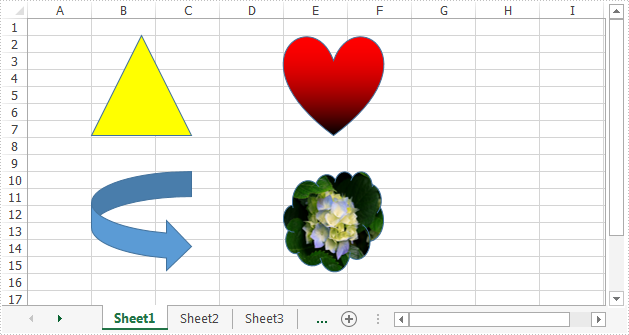Full code:

```using System.Drawing;
using Spire.Xls;
using Spire.Xls.Core;

{
class Program
{
static void Main(string[] args)
{
//Instantiate a workbook object
Workbook workbook = new Workbook();
//Get the first worksheet
Worksheet sheet = workbook.Worksheets;

IPrstGeomShape triangle = sheet.PrstGeomShapes.AddPrstGeomShape(2, 2, 100, 100, PrstGeomShapeType.Triangle);
//Fill the triangle with solid color
triangle.Fill.ForeColor = Color.Yellow;
triangle.Fill.FillType = ShapeFillType.SolidColor;

IPrstGeomShape heart = sheet.PrstGeomShapes.AddPrstGeomShape(2, 5, 100, 100, PrstGeomShapeType.Heart);
//Fill the heart with gradient color
heart.Fill.ForeColor = Color.Red;

//Add an arrow shape with default color
IPrstGeomShape arrow = sheet.PrstGeomShapes.AddPrstGeomShape(10, 2, 100, 100, PrstGeomShapeType.CurvedRightArrow);

IPrstGeomShape cloud = sheet.PrstGeomShapes.AddPrstGeomShape(10, 5, 100, 100, PrstGeomShapeType.Cloud);
//Fill the cloud with custom picture
cloud.Fill.CustomPicture(Image.FromFile("Hydrangeas.jpg"), "Hydrangeas.jpg");
cloud.Fill.FillType = ShapeFillType.Picture;

//Save the file
}
}
}
```
Friday, 26 August 2016 01:15

### Adding Picture to Excel Textbox in C#, VB.NET

Written by support iceblue

Textbox in Excel is a special kind of graphic object, which allows users to add some text in it. Moreover, textbox can be filled with solid color, gradient, pattern or a picture so that it looks more attractive. This article presents how to add a picture fill to Excel textbox and adjust the position of the picture as well.

Code Snippets:

Step 1: Create a new instance of Workbook class and get the first worksheet.

```Workbook workbook = new Workbook();
Worksheet sheet = workbook.Worksheets;
```

Step 2: Add a textbox shape into the worksheet.

```ITextBoxShape shape = sheet.TextBoxes.AddTextBox(2, 2, 200, 300);
```

Step 3: Fill the textbox with a 160x160 pixels picture.

```shape.Fill.CustomPicture(@"C:\Users\Administrator\Desktop\logo.png");
shape.Fill.FillType = ShapeFillType.Picture;
```

Step 4: Save the file.

```workbook.SaveToFile("PicFill.xlsx", ExcelVersion.Version2013);
```

When we add a picture fill to a textbox using above code, the picture will stretch to fill the shape, like below screenshot.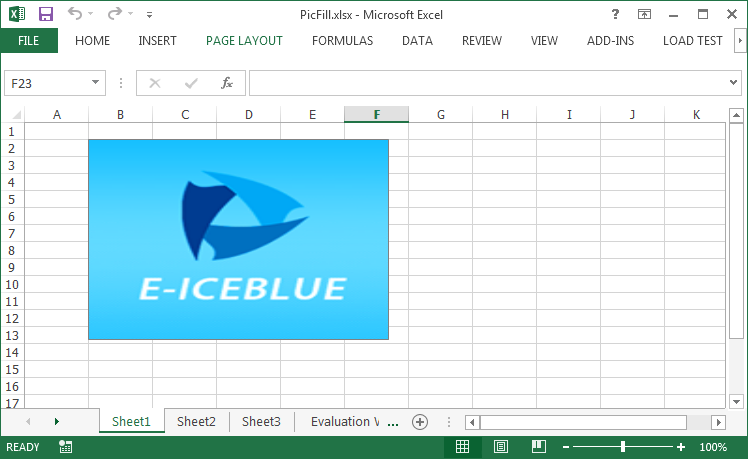If you want to prevent the image from stretching, you can adjust the size of the textbox or change the position of the picture, for example, place the image at the central position of the textbox. Following code snippet demonstrates how to center align the picture fill.

```//If the height of textbox is larger than the height of original picture, set the picture into vertical center
if (textbox.Height > textbox.Fill.Picture.Height)
{
int difH = textbox.Height - textbox.Fill.Picture.Height;
(textbox.Fill as XlsShapeFill).PicStretch.Top = difH * 100 / (textbox.Height * 2);
(textbox.Fill as XlsShapeFill).PicStretch.Bottom = difH * 100 / (textbox.Height * 2);
}
//If the width of textbox is larger than the width of original picture, set the picture into horizontal center
if (textbox.Width > textbox.Fill.Picture.Width)
{
int difW = textbox.Width - textbox.Fill.Picture.Width;
(textbox.Fill as XlsShapeFill).PicStretch.Left = difW * 100 / (textbox.Width * 2);
(textbox.Fill as XlsShapeFill).PicStretch.Right = difW * 100 / (textbox.Width * 2);
}
```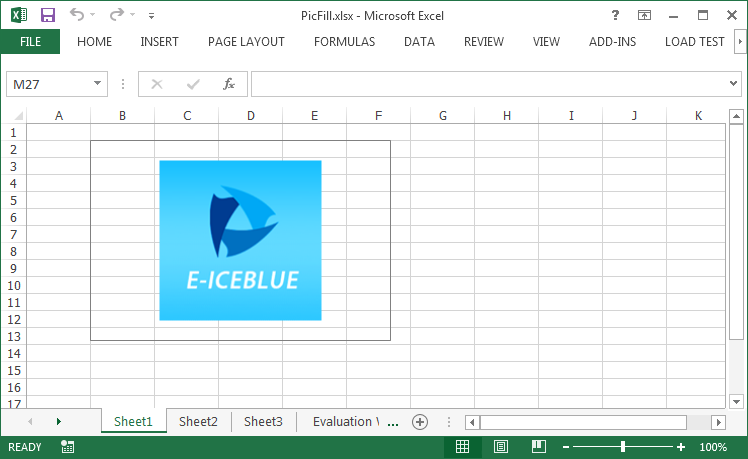Full Code:

[C#]
```Workbook workbook = new Workbook();
Worksheet sheet = workbook.Worksheets;
ITextBoxShape textbox = sheet.TextBoxes.AddTextBox(2, 2, 200, 300);

textbox.Fill.FillType = ShapeFillType.Picture;

//If the height of textbox is larger than the height of original picture, set the picture into vertical center
if (textbox.Height > textbox.Fill.Picture.Height)
{
int difH = textbox.Height - textbox.Fill.Picture.Height;
(textbox.Fill as XlsShapeFill).PicStretch.Top = difH * 100 / (textbox.Height * 2);
(textbox.Fill as XlsShapeFill).PicStretch.Bottom = difH * 100 / (textbox.Height * 2);
}
//If the width of textbox is larger than the width of original picture, set the picture into horizontal center
if (textbox.Width > textbox.Fill.Picture.Width)
{
int difW = textbox.Width - textbox.Fill.Picture.Width;
(textbox.Fill as XlsShapeFill).PicStretch.Left = difW * 100 / (textbox.Width * 2);
(textbox.Fill as XlsShapeFill).PicStretch.Right = difW * 100 / (textbox.Width * 2);
}

workbook.SaveToFile("PicFill.xlsx", ExcelVersion.Version2013);
```
[VB.NET]
```Dim workbook As New Workbook()
Dim sheet As Worksheet = workbook.Worksheets(0)
Dim textbox As ITextBoxShape = sheet.TextBoxes.AddTextBox(2, 2, 200, 300)

textbox.Fill.FillType = ShapeFillType.Picture

'If the height of textbox is larger than the height of original picture, set the picture into vertical center
If textbox.Height > textbox.Fill.Picture.Height Then

Dim difH As Integer = textbox.Height - textbox.Fill.Picture.Height

TryCast(textbox.Fill, XlsShapeFill).PicStretch.Top = difH* 100 / (textbox.Height* 2)

TryCast(textbox.Fill, XlsShapeFill).PicStretch.Bottom = difH* 100 / (textbox.Height* 2)
End If
'If the width of textbox is larger than the width of original picture, set the picture into horizontal center
If textbox.Width > textbox.Fill.Picture.Width Then

Dim difW As Integer = textbox.Width - textbox.Fill.Picture.Width

TryCast(textbox.Fill, XlsShapeFill).PicStretch.Left = difW* 100 / (textbox.Width* 2)

TryCast(textbox.Fill, XlsShapeFill).PicStretch.Right = difW* 100 / (textbox.Width* 2)
End If

workbook.SaveToFile("PicFill.xlsx", ExcelVersion.Version2013)
```
Thursday, 03 September 2015 02:46

### How to Extract Text from a Textbox on Excel worksheet in C#

Written by support iceblue

Spire.XLS supports to work with Text Box. We have already shown to you how to add the textbox to an Excel worksheet and set the font and background for the textbox. We may need to extract the value (text) from a Textbox that exists on a spreadsheet to get the abstract and introduce information for the Excel worksheet. This article will demonstrate how to extract text from Excel Textbox in C#. Check the original excel file with the textbox firstly: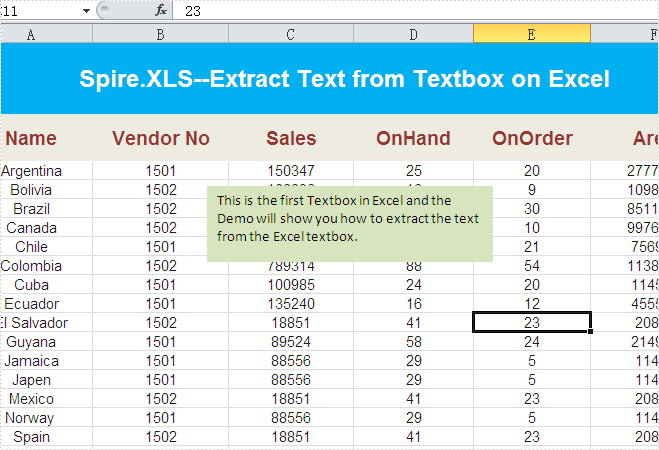Here comes to the steps of how to get the text from the excel textbox:

Step 1: Create a new instance of workbook and load an Excel file with textbox from file.

```Workbook workbook = new Workbook();
```

Step 2: Get the worksheet named in "Sheet1" which contains textbox.

```Worksheet sheet = workbook.Worksheets["Sheet1"];
```

Step 3: Get the first textbox.

```XlsTextBoxShape shape = sheet.TextBoxes as XlsTextBoxShape;
```

Step 4: Read the text from the textbox and output it.

```Console.WriteLine(shape.Text);
```

Effective screenshot: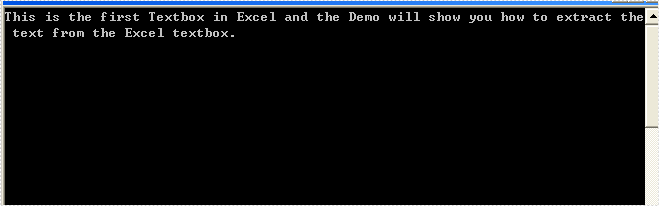Full codes:

```Workbook workbook = new Workbook();

Worksheet sheet = workbook.Worksheets["Sheet1"];
XlsTextBoxShape shape = sheet.TextBoxes as XlsTextBoxShape;

Console.WriteLine(shape.Text);
```
Thursday, 25 June 2015 02:41

### How to Insert OLE Object in Excel in C#, VB.NET

Written by support iceblue

OLE object is used to make content created in one program available in another program, for instance, we can insert Word as OLE object in Excel sheet.

As a robust component, Spire.XLS supports to insert Word and PowerPoint slide as linked object or embedded object into Excel. In this article, we make an example to explain how to insert Word as OLE object into Excel using Spire.XLS and Spire.Doc. Before coding, you need to download Spire.Office and reference related the Dlls in your VS project.

Code Snippet:

Step 1: Define a GetDocImage(string doxcFile) method to get olePicture. Actually, the olePicture is an image of data information in original Word document. The image generated from the specified page will be shown in Excel sheet after inserting OLE object into it.

```private static Image GetDocImage(string docxFile)
{
Document document = new Document();
return document.SaveToImages(0, Spire.Doc.Documents.ImageType.Bitmap);

}
```

Step 2: Insert OLE object in Excel. After getting the worksheet from Excel file, we call GetDocImage(string doxcFile) method which is defined in the first step to get image source and then use the ws.OleObjects.Add(string FileName, Image image, OleLinkType linkType) method to insert the new OLE object to worksheet.

```static void Main(string[] args)
{
Workbook workbook = new Workbook();
Worksheet ws = workbook.Worksheets;
//insert OLE object
string docx = "d:\\sample.docx";
Image image = GetDocImage(docx);
oleObject.Location=ws.Range["B4"];
oleObject.ObjectType = OleObjectType.WordDocument;
//save the file
workbook.SaveToFile("result.xlsx",ExcelVersion.Version2010);
System.Diagnostics.Process.Start("result.xlsx");
}
```

Result: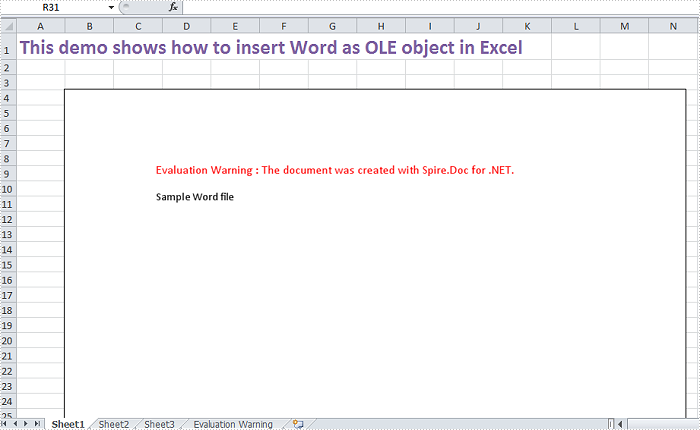Full Code:

[C#]
```private static Image GetDocImage(string docxFile)
{
Document document = new Document();
return document.SaveToImages(0, Spire.Doc.Documents.ImageType.Bitmap);

}
static void Main(string[] args)
{
Workbook workbook = new Workbook();
Worksheet ws = workbook.Worksheets;
//insert OLE object
string docx = "d:\\sample.docx";
Image image = GetDocImage(docx);
oleObject.Location=ws.Range["B4"];
oleObject.ObjectType = OleObjectType.WordDocument;
//save the file
workbook.SaveToFile("result.xlsx",ExcelVersion.Version2010);
System.Diagnostics.Process.Start("result.xlsx");
}
```
[VB.NET]
```Private Shared Function GetDocImage(docxFile As String) As Image
Dim document As New Document()
Return document.SaveToImages(0, Spire.Doc.Documents.ImageType.Bitmap)

End Function
Private Shared Sub Main(args As String())
Dim workbook As New Workbook()